The standard deviation of a set of data gives an indication of the amount of dispersion,of members of set from the measure of central tendency, of a given set of discrete data. Its value is the root-mean-square value of the members of the set. Below are steps to follow, when calculating the standard deviation of a Discrete data.

Step 1: Determine the measure of central tendency, usually the mean value, (occasionally the median or modal values are specified),

Step 2 : Calculate the deviation of each member of the set from the mean,

Step 3: Calculate the square of the deviation of each member set.

Step 4: Calculate the product of the frequency and the square of the mean deviations obtained from the data sets, if it is a Discrete data.

Example: The frequency distribution for the values of test score in a class of 48 student is as shown below. Calculate the standard deviation from the mean test score , correct to 3 significant figures.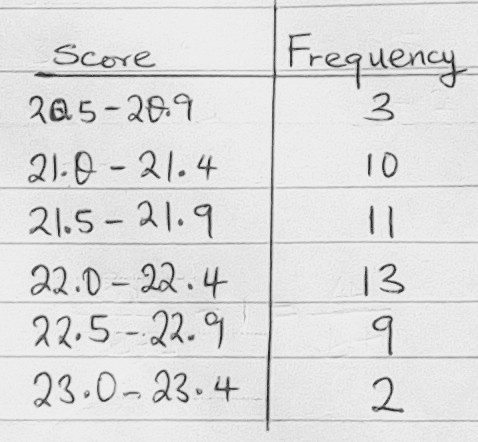Step 1: Determine the mean value (x̅) of the given set of data. The mean for a group of data, like the one above can be calculated using the formula on the image below.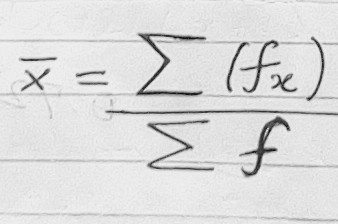where f is the class frequency and x is the class midpoint value. Below is a table of the class frequency and class midpoint values for the above set of data.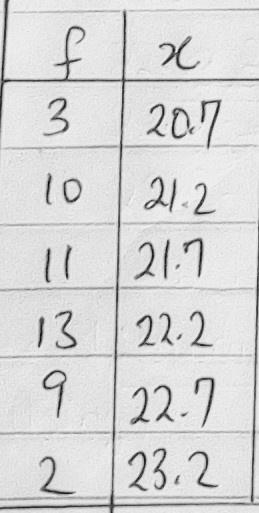View pictures in App save up to 80% data.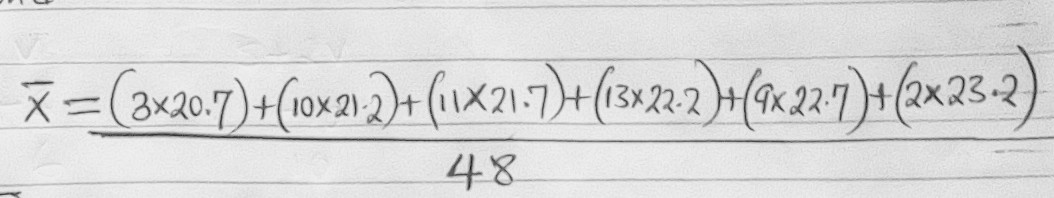View pictures in App save up to 80% data.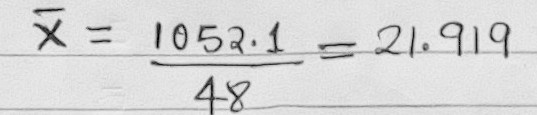View pictures in App save up to 80% data.

The mean value is 21.92 , correct to 4 significant figures.

Step 2 : Calculate the standard deviation from the mean test score. The standard deviation can be calculated using the formula on the image below, if the mean of the set of data is known.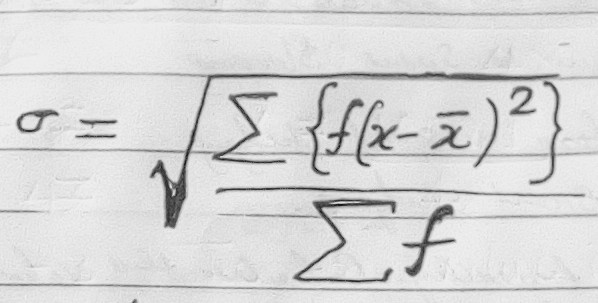View pictures in App save up to 80% data.x is the midpoint value of the class and  is the calculated mean, which is 21.92. In order to save time, the solution to the standard deviation has been Presented in the following images .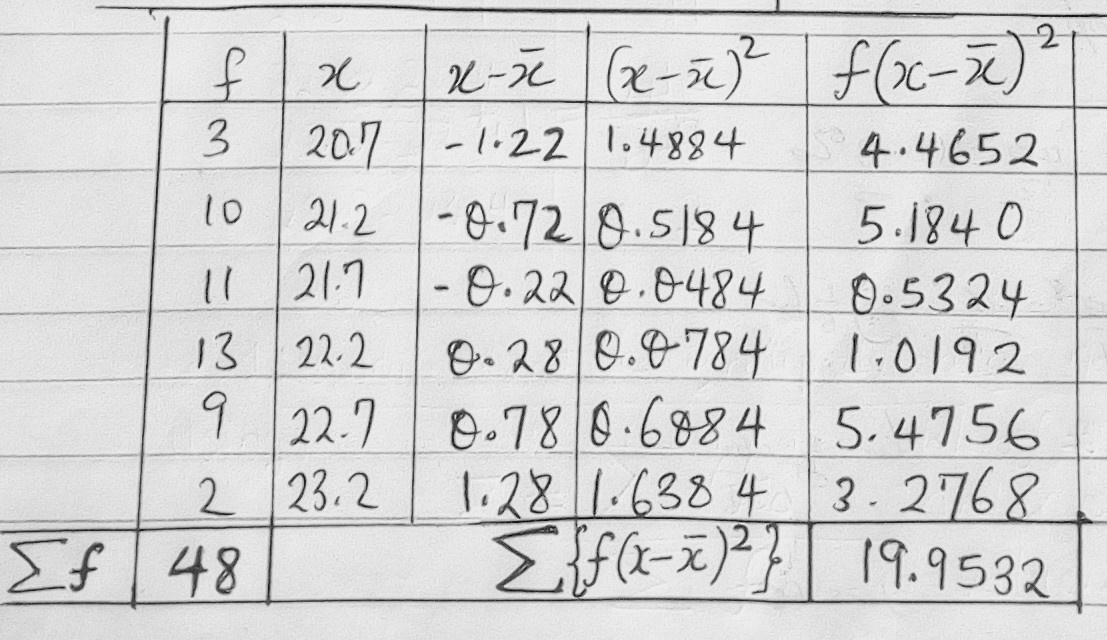View pictures in App save up to 80% data.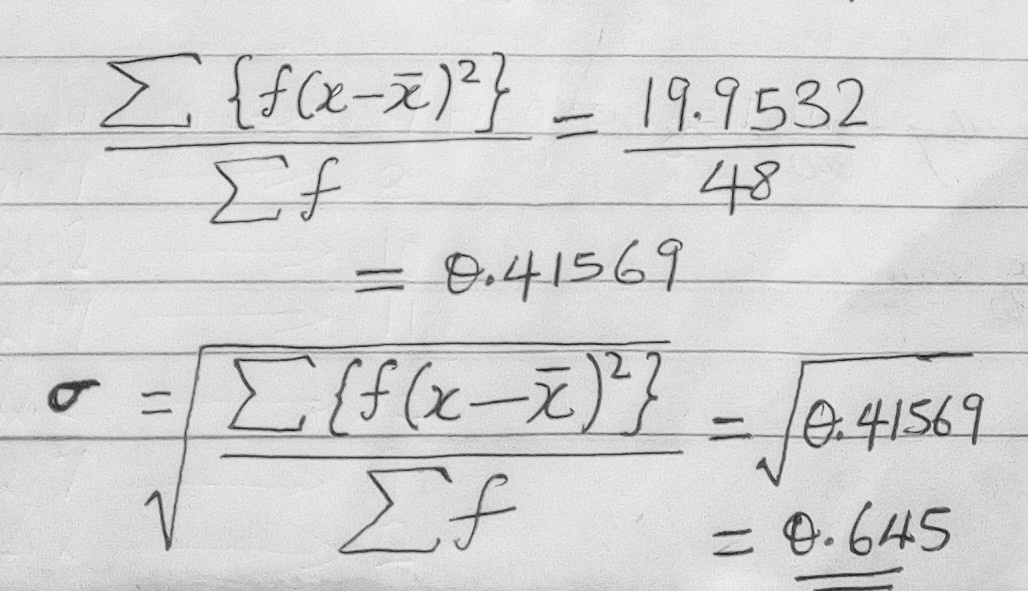View pictures in App save up to 80% data.

A Venn diagram can be used to show the relationship between the outcomes of a random events, the sample space and events associated with the outcomes. The Venn diagram in the image below shows the difference between the universal set, a sample space and events and outcomes as subsets of the sample space.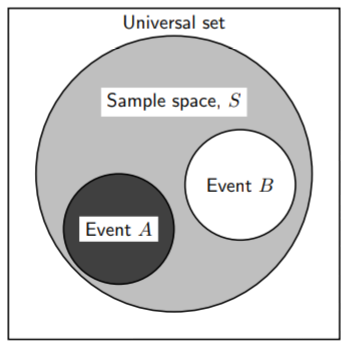View pictures in App save up to 80% data.Venn diagrams can also be used to indicate the union and intersection between events in a sample space.

For example, In a box there are pieces of paper with the numbers from 1 to 9 written on them.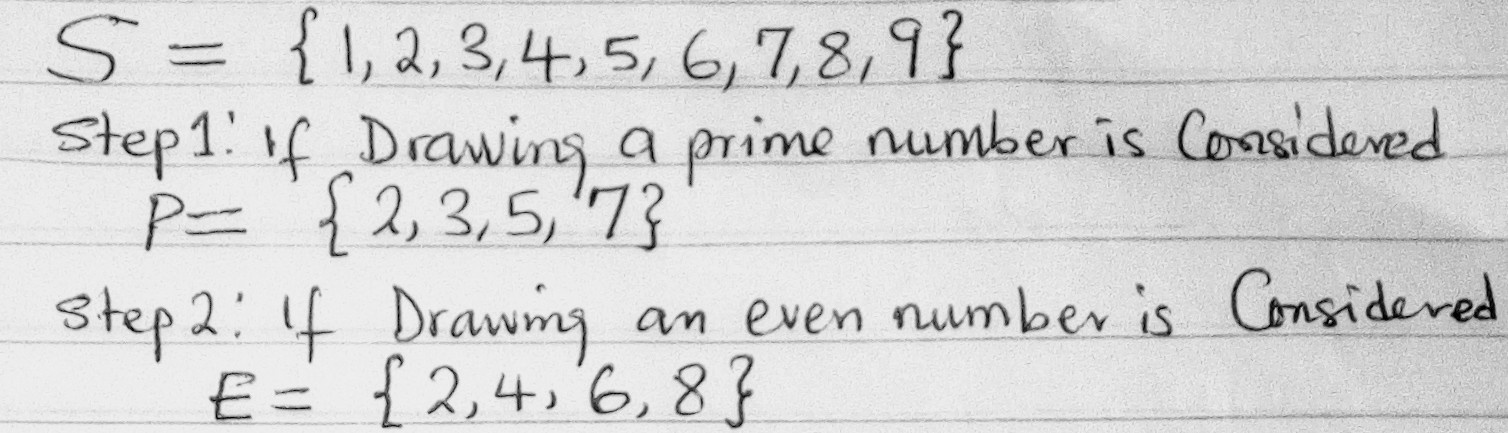View pictures in App save up to 80% data.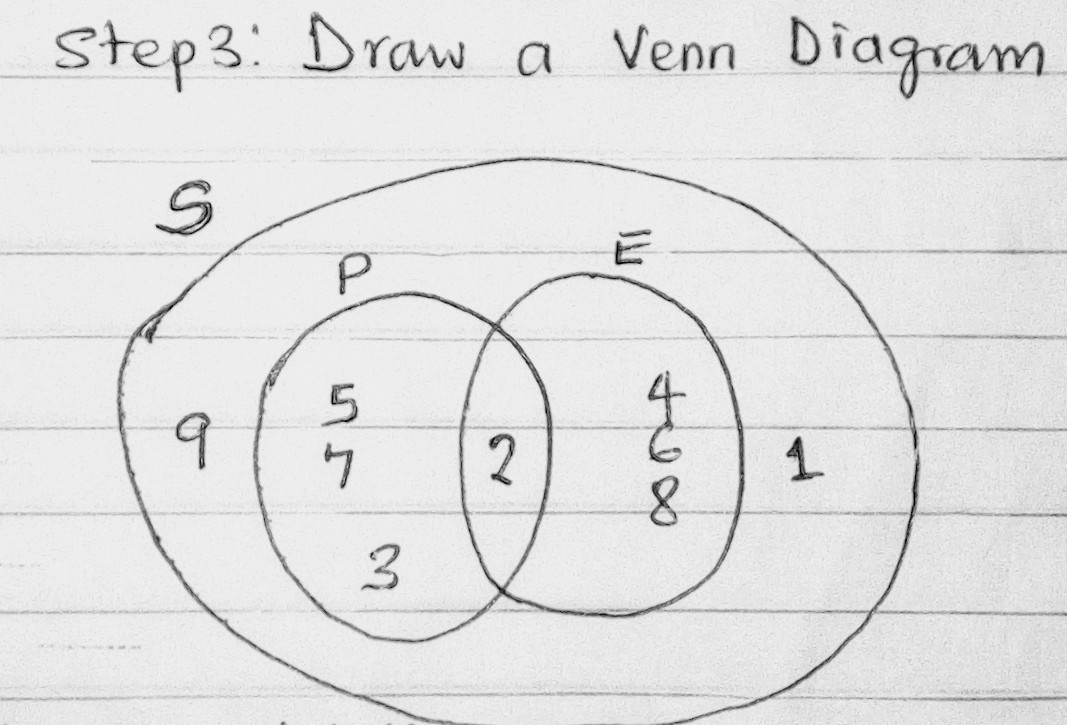View pictures in App save up to 80% data.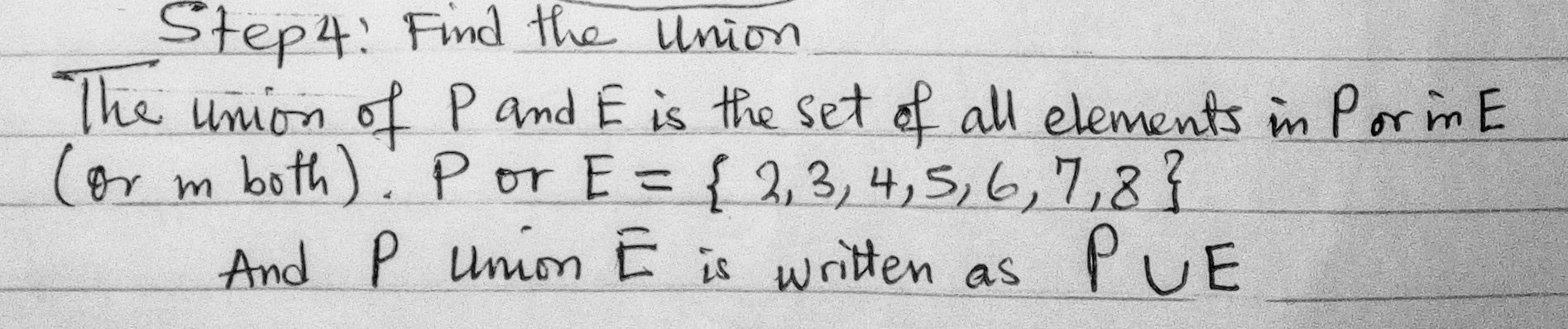View pictures in App save up to 80% data.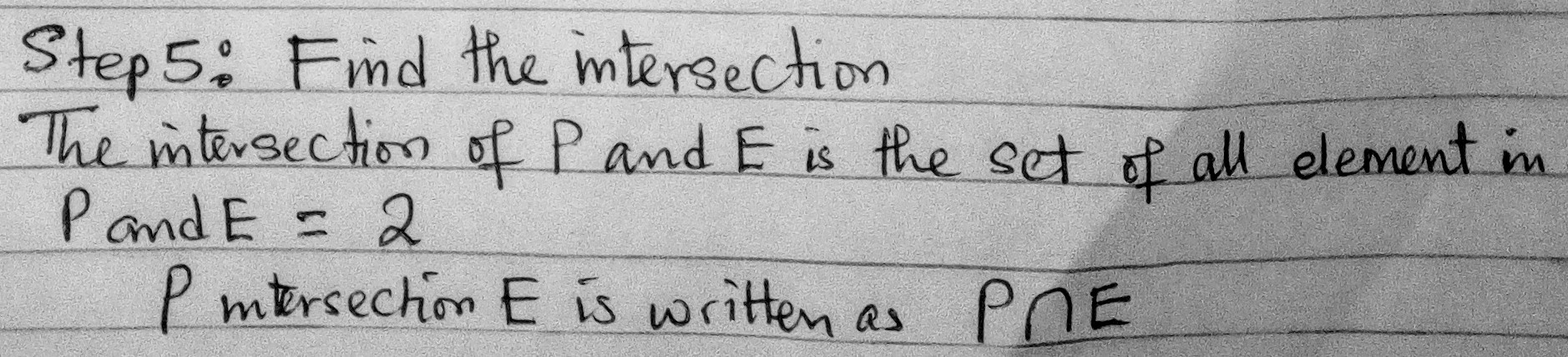View pictures in App save up to 80% data.

The word probability relates to uncertain events or knowledge, being closely related in meaning to likely, risky, hazardous, and doubtful. Chance, odds, and bet are other words expressing similar ideas. For example, a Weather forecast might predict that there is a 60% chance that it will rain tomorrow in your State. This means that in 6 of every 10 times when your state or city is in that current weather condition, it has always rain, And there is also the outcome of 4 out 10 times which it did not rain. Thus, Probability is connected with uncertainty.

## Classical Theories of Probability

1. Equally likely outcomes are outcomes which have an equal chance of happening. For example when a fair coin is tossed, each outcome in the sample space S = headstails is equally likely to occur.

2. When all the outcomes are equally likely (in any activity), you can calculate the probability of an event happening by using the following definition: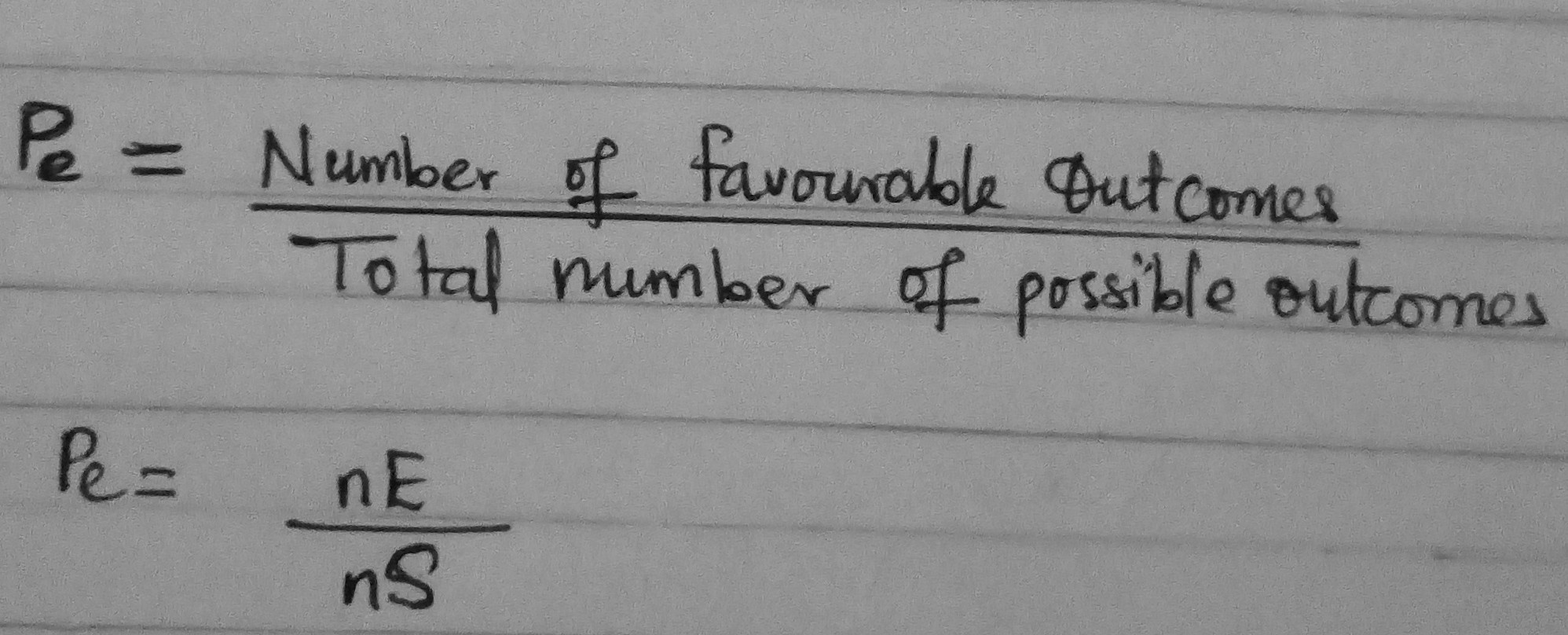View pictures in App save up to 80% data.

For example, when you throw a fair dice the possible outcomes are S = {1,2,3,4,5,6} i.e the total number of possible outcomes n(S)=6.

Event 1: get a 4; The only possible outcome is a 4, i.e E=4 i.e number of favourable outcomes: n(E)=1.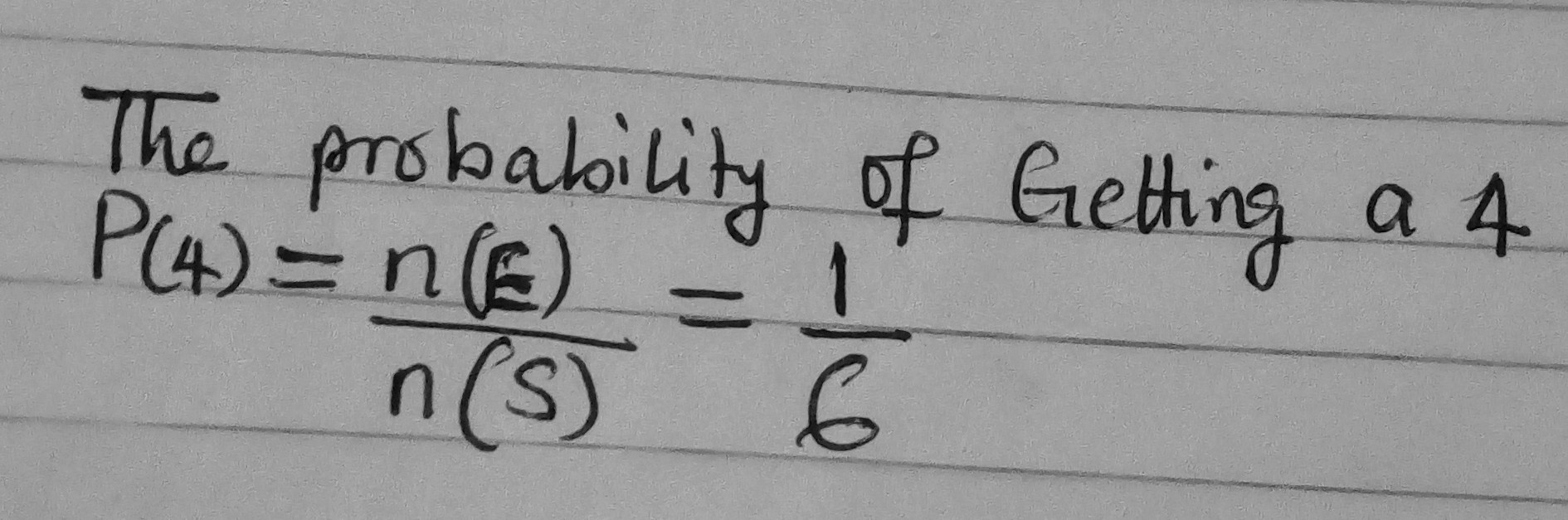View pictures in App save up to 80% data.

Event 2: get a number greater than 3; the Favourable outcomes: E = {4,5,6}, Number of favourable outcomes: n(E)=3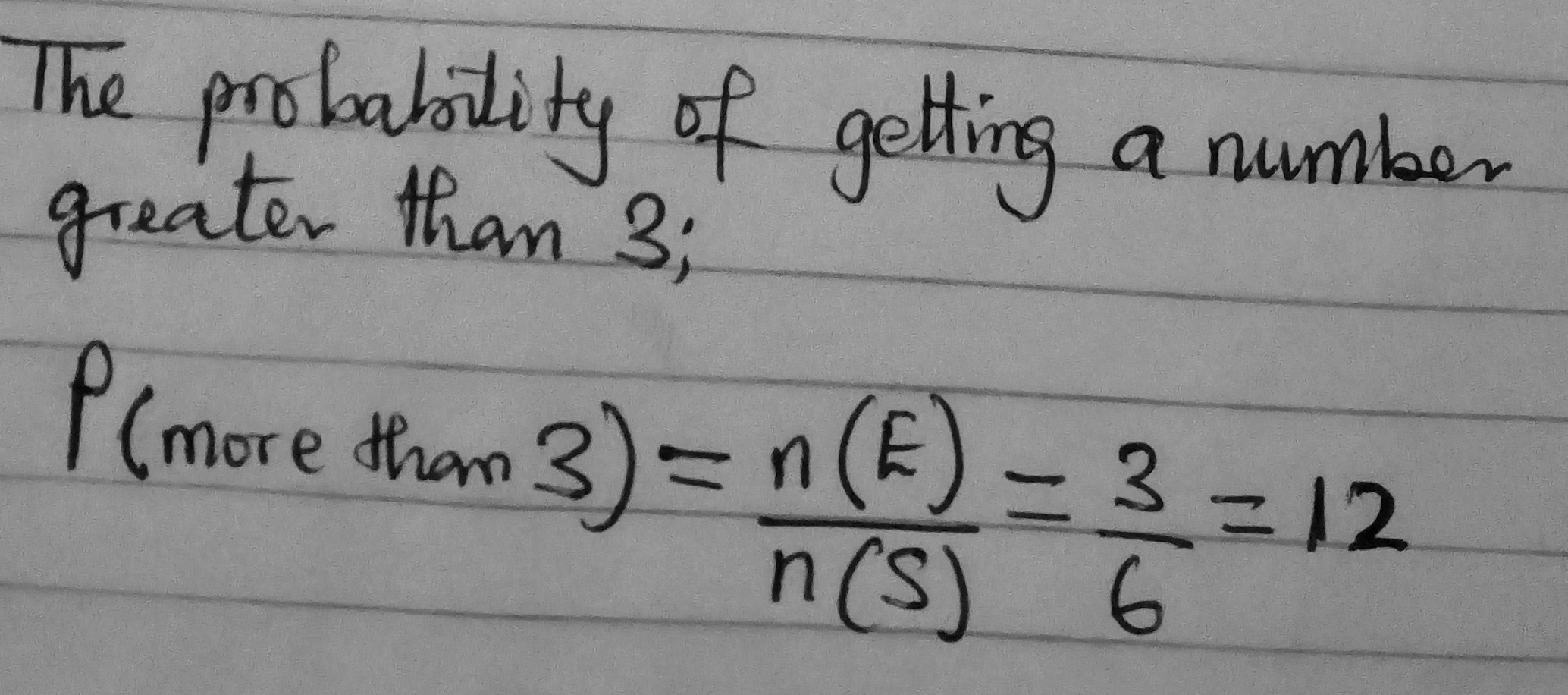View pictures in App save up to 80% data.

Various probabilities relating to a deck of cards.

A standard deck of cards (without jokers) has 52 cards. If we randomly draw a card from the deck, we can think of each card as a possible outcome. Therefore,there are 52 total outcomes. We can now look at various events and calculate their probabilities:

1. Out of the 52 cards, there are 13 clubs. Therefore, if the event of interest is drawing a club, there are 13 favourable outcomes, and the probability of this event is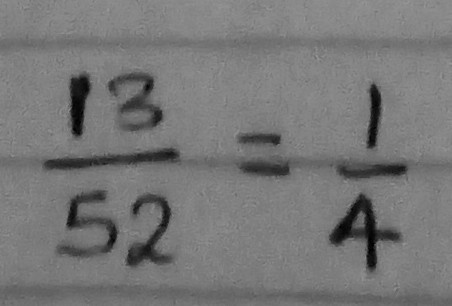View pictures in App save up to 80% data.

2. There are 4 kings (one of each suit). The probability of drawing a king is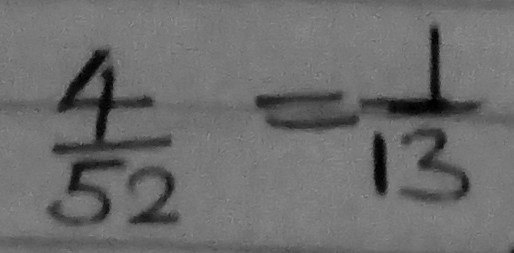View pictures in App save up to 80% data.

3. What is the probability of drawing a king OR a club? This example is slightly more complicated. We cannot simply add together the number of number of outcomes for each event separately (4 + 13 = 17) as this inadvertently counts one of the outcomes twice (the king of clubs).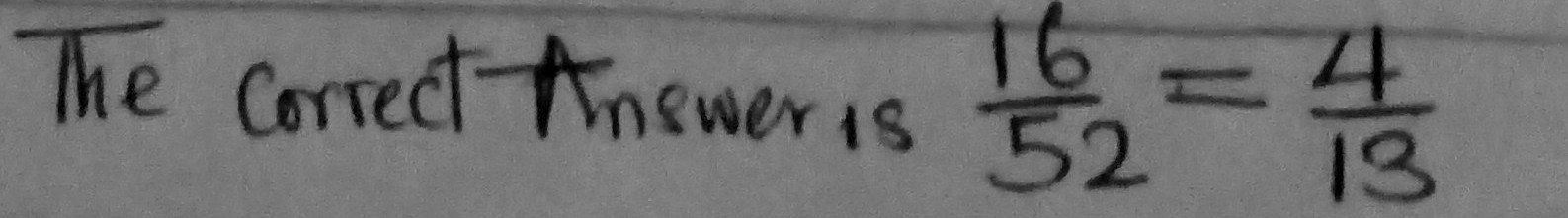View pictures in App save up to 80% data.

Probability Identities; The image below apply to probabilities, for a sample space S and two events and B, within S.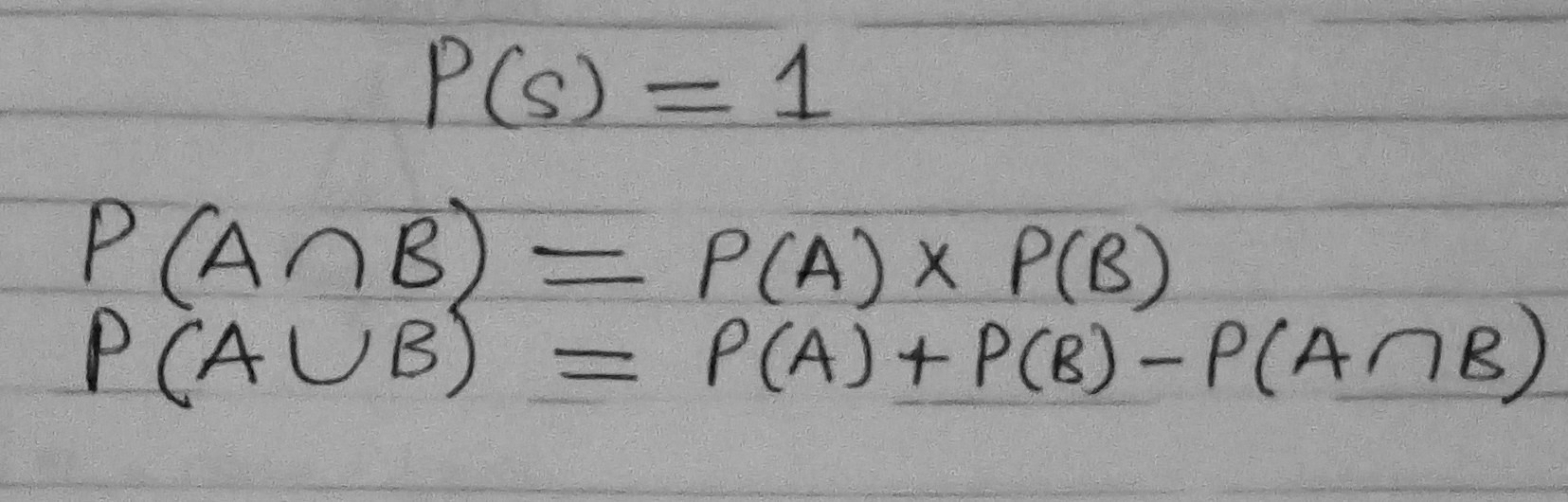View pictures in App save up to 80% data.

Example : What is the probability of selecting a black or red card from a pack of 52 cards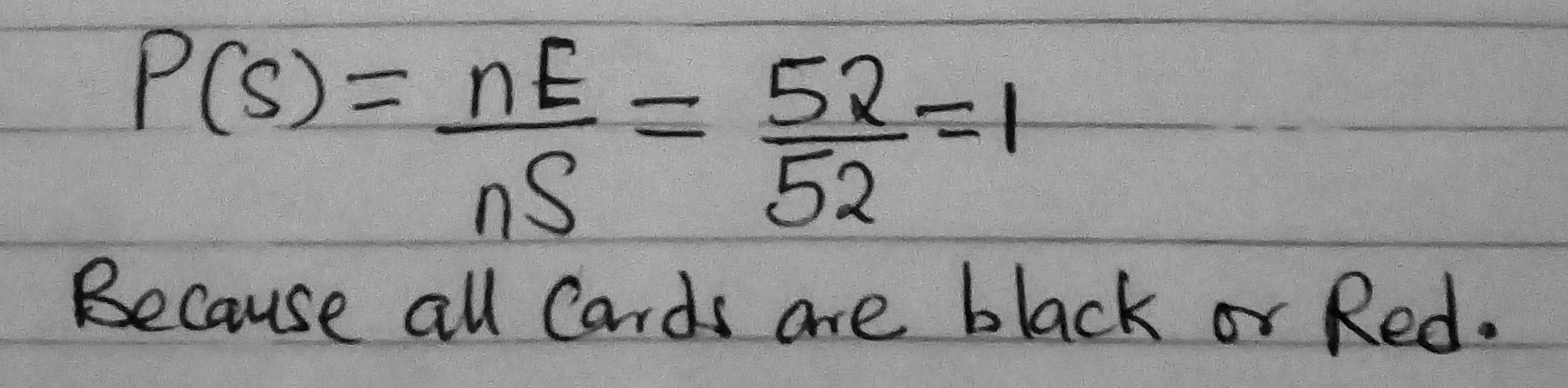View pictures in App save up to 80% data.

Example; What is the probability of drawing a club or an ace with one single pick from a pack of 52 cards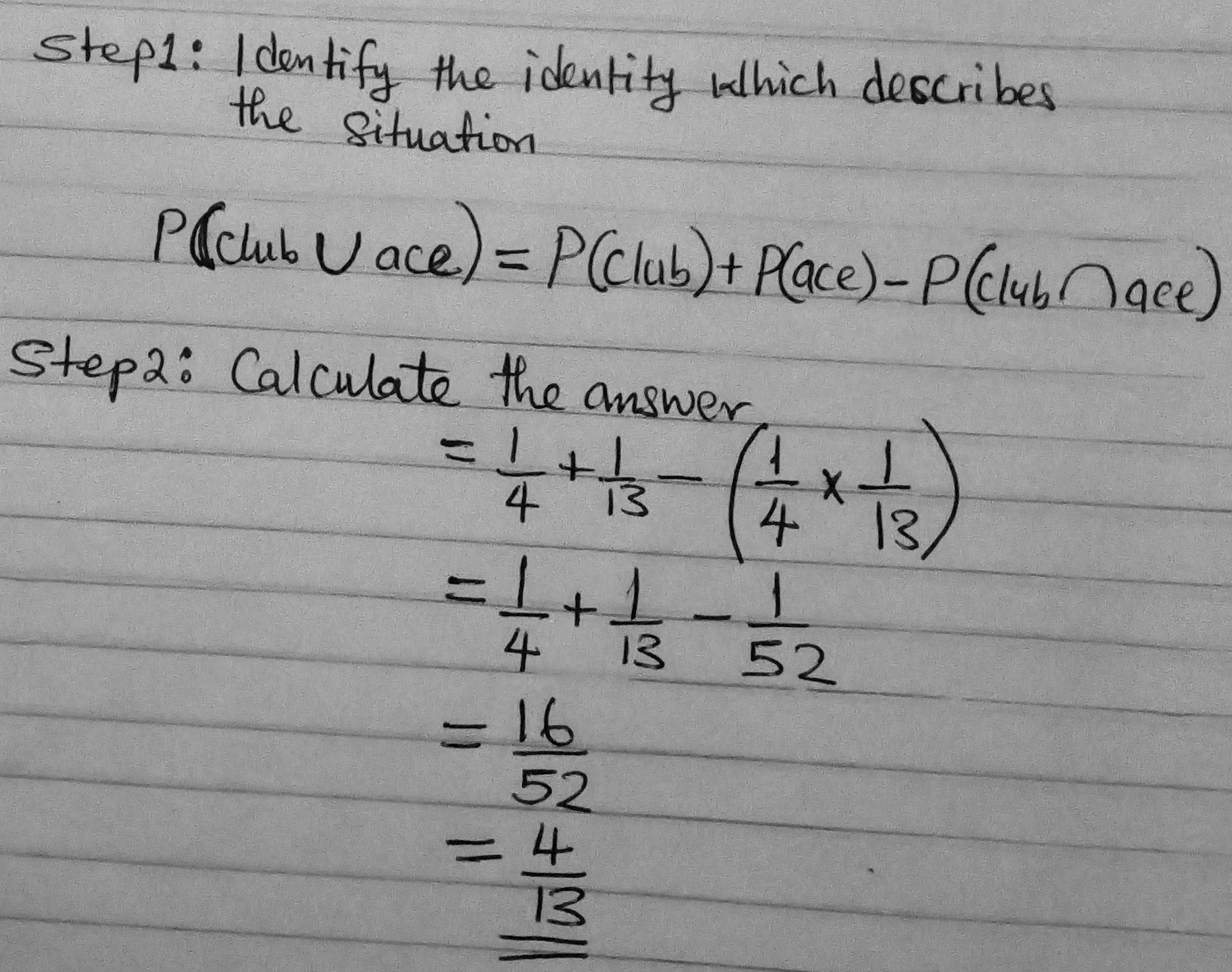View pictures in App save up to 80% data.Example ; If you throw two dice, one red and one blue, what is the probability that at least one of them will be a six?

Solution; To solve that kind of question, first work out the probability that there will be no six.

The probability that the red dice will not be a six is 5/6, and that the blue one will not be a six is also 5/6.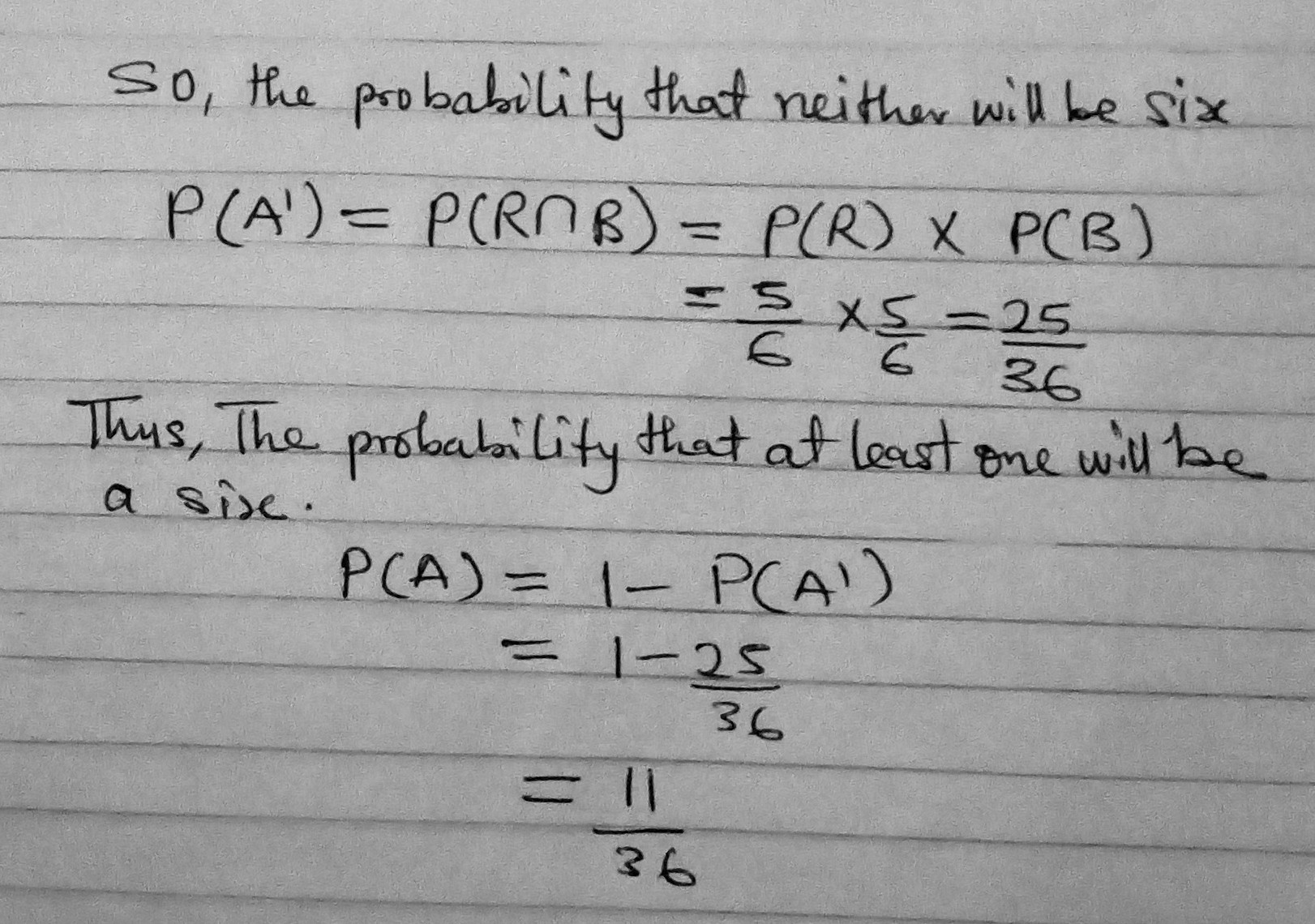View pictures in App save up to 80% data.

Example ; A bag contains three red balls, five white balls, two green balls and four blue balls:

I. Calculate the probability that a red ball will be drawn from the bag.

II. Calculate the probability that a ball which is not red will be drawn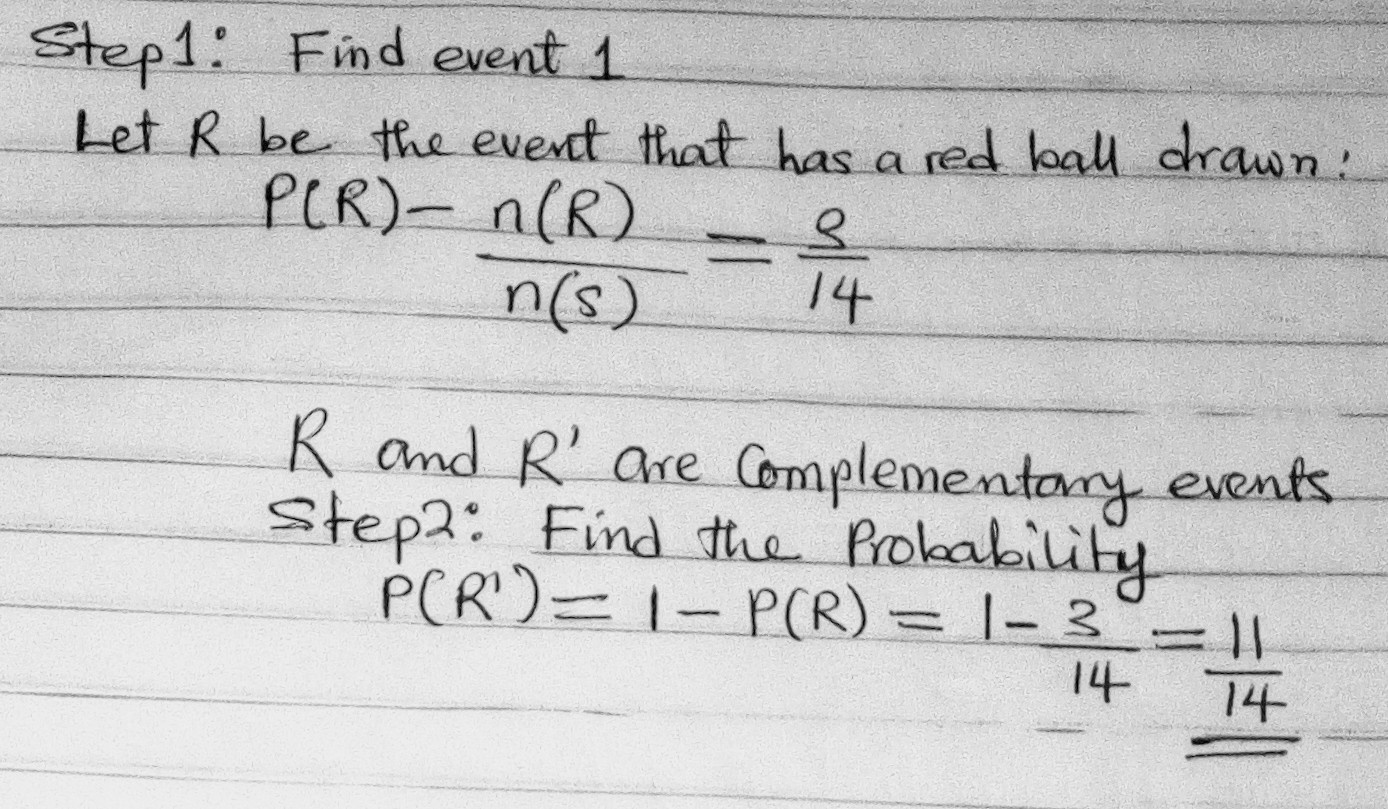View pictures in App save up to 80% dat